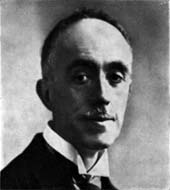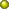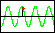# WAVE MECHANICS

### Prince Louis de Broglie

óóóóó(   1892-1987   )óóóóó

In 1923, while still a graduate student at the University of Paris, Louis de Broglie published a brief note in the journal Comptes rendus containing an idea that was to revolutionize our understanding of the physical world at the most fundamental level.   He had been troubled by a curious "contradiction" arising from Einstein's special theory of relativity.

First, he assumed that there is always associated with a particle of mass $m$ a periodic internal phenomenon of frequency $\nu \text{.}$   For a particle at rest, he equated the rest mass energy $m{c}^{2}$ to the energy of the quantum of the electromagnetic field $h\nu \text{.}$ That is, $m{c}^{2}=h\nu \text{,}$ where $h$ is Planck's constant and $c$ is the speed of light.

De Broglie noted that relativity theory predicts that, when such a particle is set in motion, its total relativistic energy will increase, tending to infinity as the speed of light is approached.   Likewise, the period of the internal phenomenon assumed to be associated with the particle will also increase (due to time dilation).   Since period and frequency are inversely related, a period increase is equivalent to a decrease of frequency and, hence, of the energy given by the quantum relation $h\nu \text{.}$   It was this apparent incompatibility between the tendency of the relativistic energy to increase and the quantum energy to decrease that troubled de Broglie.1 [footnote]

The manner in which de Broglie resolved this apparent contradiction is the subject of the famous 1923 Comptes rendus note [Comptes rendus de l'Acadťmie des Sciences, vol. 177, pp. 507-510 (1923)].   The original note in French and an English translation are available here by kind permission of the Acadťmie des Sciences and the Fondation Louis de Broglie.   The assistance of Professor Sophie Papaefthymiou of the University of Paris in obtaining permission to post these materials is gratefully acknowledged.

## 1923 Comptes rendus Note

De Broglie's 1923 Comptes rendus note is available here as follows:English translation as a web page,English translation in Adobe PDF format, andFacsimile of the original French in Adobe PDF format.

## Phase Wave Animation

For an illustration of the relationship between the phase wave proposed by de Broglie and the instantaneous state of the internal periodic phenomenon de Broglie assumed to be associated with a particle, see the accompanying animated graphic.

## Further Details

De Broglie presented a more detailed exposition of the ideas contained in his 1923 note in the first chapter of his doctoral thesis Recherches sur la thťorie des Quanta (University of Paris, 1924).   An English translation can be found in "Phase Waves of Louis deBroglie", Am. J. Phys. vol. 40 no. 9, pp. 1315-1320, September, 1972.   The ideas are also revisited in the first chapter of de Broglie's book Non-linear Wave Mechanics: A Causal Interpretation, Elsevier Publishing Company, 1960.

óóóóóóóóóó
1 A consequence of de Broglie's reasoning is that a phase wave, often referred to as the "pilot" wave, appears to accompany the particle.   This is made evident in de Broglie's 1923 Comptes rendus note.   Yet, modern introductions to quantum mechanics often fail to emphasize that this phase wave arises as an inevitable consequence of de Broglie's assumption of the internal periodic phenomenon of the particle and the transformation laws of the special theory of relativity.   A notable exception is "Introduction to Quantum Mechanics," A. P. French and Edwin F. Taylor, W. W. Norton & Co., pp. 55-62 (1978).

Given de Broglie's assumptions, quantum mechanics, which is to say the study of the behavior and interpretation of the phase wave, is the study of an inherently relativistic phenomenon.   In this sense, if it were not for relativistic effects, quantum (wave) mechanics would not exist!   Yet, the phrase "non-relativistic quantum mechanics" is a commonplace.   Clearly, this phrase should be understood to refer to the quantum mechanical description of particles moving at speeds very much less than the speed of light, but not to imply that relativistic effects are ever of no consequence in quantum mechanics.

An example is provided by the orbits of the electron in the hydrogen atom.   Even for the innermost orbits, the speed of the electron is very much less than the speed of light.   While the motion of the electron is, therefore, "non-relativistic" in the sense that the relativistic corrections to the classically (non-quantum mechanical) predicted behavior of the electron would be inconsequential, relativistic effects in fact dominate.   That is, the phase wave associated with the electron, which leads directly to the quantization of the allowed orbits, is, according to de Broglie's model, relativistic in origin.   If not for the relativistic transformations that give rise to the phase wave, quantization of the orbits and, hence, the spectrum of the hydrogen atom would not occur.   Clearly then, relativistic effects cannot be considered to be inconsequential in determining the electronic properties of the hydrogen atom, even though the speed of the orbiting electron is very much less than the speed of light. [return from footnote]